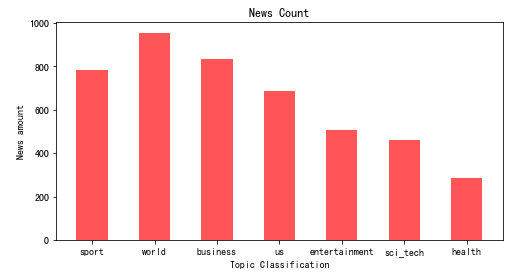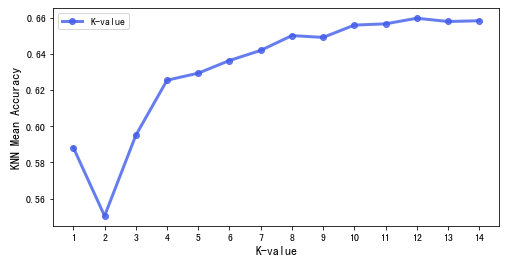• 文章目录柱状图折线图 柱状图 在柱状图时，横坐标上的文字可能会重叠，我们可以根据自己的需要改变label的间距以及调节颜色的透明程度等。以下图为例： 对应的代码为： # 柱状图 import matplotlib.pyplot as plt...


文章目录
柱状图折线图

柱状图
在画柱状图时，横坐标上的文字可能会重叠，我们可以根据自己的需要改变label的间距以及调节颜色的透明程度等。以下图为例：对应的代码为：
# 柱状图
import matplotlib.pyplot as plt

# 调节x轴之间的间距
params = {
'figure.figsize': '8, 4'
}
plt.rcParams.update(params)

Y=[782,955,835,688,508,459,285]

# 0.5是柱的粗细，alpha是颜色的透明程度，可以根据自己需要调节
plt.bar(X,Y,0.5,color="red",alpha=0.6)

# 设置横纵坐标以及title
plt.xlabel("Topic Classification")
plt.ylabel("News amount")
plt.title("News Count")

plt.show()



折线图
在折线图上，有时需要我们加重数值点，可以让结果更加明显。以下图为例：对应的代码为：
# 折线图
import matplotlib.pyplot as plt

y=[0.5882,0.5504,0.595,0.6255,0.6294,0.6364,0.642,0.6502,0.6492,0.656,0.6567,0.6598,0.658,0.6584]
x=['1','2','3','4','5','6','7','8','9','10','11','12','13','14']

# ro-代表实心原点，alpha代表颜色的透明度，linewidth代码线的宽度，同时设置一个legend
plt.plot(x, y, 'ro-', color='#4169E1', alpha=0.8, linewidth=3, label='K-value')

plt.xlabel("K-value", fontsize=12)
plt.ylabel("KNN Mean Accuracy", fontsize=12)

# legend位置
plt.legend(loc="upper left")

plt.show()


展开全文• 网上发现一个讲Python柱状图和折线图的小视频，我觉得讲的超基础，很适合小白，特来分享给大家~ 如果你也想学习数据分析，跟我一起看看下方视频，听知名技术专家李刚老师对Python可视化模块详细...
Python爬虫太火了，没写过爬虫，都不敢说自己学过Python？！
可是刚一开始学我就遇到了难题----数据分析！听起来很高大上，有没有？
想要做爬虫，就得先学会使用数据分析工具，制作图表这是最基本的。网上发现一个讲Python做柱状图和折线图的小视频，我觉得讲的超基础，很适合小白，特来分享给大家~
如果你也想学习数据分析，跟我一起看看下方视频，听知名技术专家李刚老师对Python可视化模块详细解析，
李刚老师出版的《疯狂Java》系列图书曾得到市场的广泛认可，经过多次再版，已被多家高校选作教材。上方视频来自于李刚老师的在线视频课程《21天通关Python》第九章 数据分析
鉴于大家都有学习Python的困惑，今天就给大家推荐一本巨有影响力的Python实战书，上线时间仅2个月，就超越了众多实力派，成京东和当当网上的长期畅销图书，并且收获了3.4W的五星好评。
这本书可谓是笔者独家私藏图书之一了，对我学习Python有着莫大的帮助，在京东上也常常"断货"，这次拿出来给大家分享一下,希望能帮到大家。
《21天通关Python》视频课程以畅销图书为教材，由图书作者李刚亲自操刀讲解；上手门槛低，可作为0基础掌握Python教材；书籍+线上复合型学习场景特别适合Python小白学习！
点击查看课程： https://edu.csdn.net/bundled/detail/49?utm_source=jiansuopy62_1 （含图书邮寄+视频教程+社群答疑+导师带队）
笔者跟大家分享一个福利！下单时输入优惠码csdn66，立减20元，券后仅需99元！
扫码入Python技术交流群，可免费听技术讲座+领学习资料+视频课免费看！展开全文学习编程
• 网上发现一个讲Python柱状图和折线图的小视频，我觉得讲的超基础，很适合小白，特来分享给大家~如果你也想学习数据分析，跟我一起看看下方视频，听知名技术专家李刚老师对Python可视化模块详细解析，...
Python爬虫太火了，没写过爬虫，都不敢说自己学过Python？！
可是刚一开始学我就遇到了难题----数据分析！听起来很高大上，有没有？
想要做爬虫，就得先学会使用数据分析工具，制作图表这是最基本的。网上发现一个讲Python做柱状图和折线图的小视频，我觉得讲的超基础，很适合小白，特来分享给大家~
如果你也想学习数据分析，跟我一起看看下方视频，听知名技术专家李刚老师对Python可视化模块详细解析，
Python 数据分析
李刚老师出版的《疯狂Java》系列图书曾得到市场的广泛认可，经过多次再版，已被多家高校选作教材。上方视频来自于李刚老师的在线视频课程《21天通关Python》第九章 数据分析
鉴于大家都有学习Python的困惑，今天就给大家推荐一本巨有影响力的Python实战书，上线时间仅2个月，就超越了众多实力派，成京东和当当网上的长期畅销图书，并且收获了3.4W的五星好评。
这本书可谓是笔者独家私藏图书之一了，对我学习Python有着莫大的帮助，在京东上也常常"断货"，这次拿出来给大家分享一下,希望能帮到大家。
《21天通关Python》视频课程以畅销图书为教材，由图书作者李刚亲自操刀讲解；上手门槛低，可作为0基础掌握Python教材；书籍+线上复合型学习场景特别适合Python小白学习！
笔者跟大家分享一个福利！下单时输入优惠码csdn66，立减20元，券后仅需99元！
扫码入Python技术交流群，可免费听技术讲座+领学习资料+视频课免费看！展开全文• from matplotlibimport pyplotimport randomx = list(range(0,100))y = [random.randint(0,100) for r in range(0,100)]fig1 = pyplot.figure()#初始化一个空白画布...)#生成一个折线图，X轴，Y轴，图形样式pyplo...
from matplotlib
import pyplot
import random
x = list(range(0,100))
y = [random.randint(0,100) for r in range(0,100)]
fig1 = pyplot.figure()#初始化一个空白画布
pyplot.plot(x, y, "-")#生成一个折线图，X轴，Y轴，图形样式
pyplot.title("First Plot – Random integers’)
pyplot.xlabel("X Axis’)
pyplot.ylabel("Y Axis’)
pyplot.show()
|
生成的图片见下图：生成的随机数折线图
结合CSV文件生成图形
CSV文件如下图：csv 数据
该数据可以由Arduino生成，参考下文：
Python与arduino文件IO操作简介
该例子将生成两个图片，一个是折线图一个是柱状图，代码如下：
import csv
from matplotlib
import pyplot
num =
[]
btnValues =
[]
potValues =
[]
with open("Arduino_data.csv’, "r’) as f:
num.append(int(row))#序列
potValues.append(float(row))#电位计数据列
btnValues.append(int(row))#按钮数据列
pyplot.subplot(2, 1, 1)##三个参数的意思是：整个图表分为2行1列，该子图表位于第一行
pyplot.plot(num, potValues, "-")#生成折线图
pyplot.title("Line plot – " + header)
pyplot.xlim([1, 30])
pyplot.xlabel("X Axis’)
pyplot.ylabel("Y Axis’)
pyplot.subplot(2, 1, 2)#三个参数的意思是：整个图表分为2行1列，该子图表位于第二行
pyplot.bar(num, btnValues)#生成柱状图
pyplot.title("Bar chart – " + header)
pyplot.xlim([1, 30])#x轴坐标范围
pyplot.xlabel("X Axis’)
pyplot.ylabel("Y Axis’)
pyplot.tight_layout()#下面有比较
pyplot.show()
|有pyplot.tight_layout()语句无pyplot.tight_layout()语句
喜欢文章，欢迎大家转发！！！
|
展开全文• 今日给大家分享一个Python读取Excel表格，同时采用表格中的数值画图柱状图和折线图，这里只需要几行代码便可以实。首先我们需要安装一个Excel操作的库xlrd，这个很简单，在安装Python后直接在DOS命令下输入pip ...
• # 画柱状图 plt.bar(x_labels, grid) 画折线图 """ for i in range(len(grid)-1): plt.plot([x_labels[i],x_labels[i+1]],[grid[i],grid[i]],color = 'b') plt.plot([x_labels[i],x...
• 1. 背景Python在一些数据可视化的过程中需要使用 plt 函数柱状图和折线图。2. 导入import matplotlib.pyplot as plt3. 柱状图array= np.array(array)plt.hist(array, bins=50,facecolor="red", edgecolor="red" ,...
• 先看画折线图观察两组数据的关联关系我们举例，有两组数据，一组数据是dgp一组是对应的年份。那么我们可以出年份与GDP的关系图，是上升还是下降来来来看代码。#-*-coding:utf-8-*-#******************************...
• /usr/bin/python3from pyecharts.charts import Bar, Pagefrom pyecharts import options as optsx = [ ‘python数据可视化库 a1‘,‘python数据可视化库 b1‘,‘python数据可视化库 c1‘ ]y1 = [ 1140,520,270 ]y2...
• Python在一些数据可视化的过程中需要使用 plt 函数柱状图和折线图。 2. 导入 import matplotlib.pyplot as plt 3. 柱状图 array= np.array(array) plt.hist(array, bins=50,facecolor="red", ...
• Python matplotlib：使用matplotlib绘制--柱状图和折线图的组合。废话不多说，直接上效果图和代码。代码如下:#柱状图import numpy as npimport matplotlib.pyplot as pltC1 = '#99CCFF'#C2 = '#CCFFFF'C2 = '#CCCCFF...
• 今日给大家分享一个python读取excel表格，同时采用表格中的数值画图柱状图和折线图，这里只需要几行代码便可以实。首先我们需要安装一个excel操作的库xlrd，这个很简单，在安装python后直接在dos命令下输入pip ...
• 记录一下这两天画图遇到的问题解决方法 pythion版本3.5，调用的是matplotlib. plt
• 利用python可以对数据进行可视化分析，这里展示两种常见的图形，分别是柱状图和折线图。 欢迎大家转发，一起传播知识和正能量，帮助到更多人。辛苦大家转发时注明出处(也是咱们公益编程交流群的入口网址)，刘...
• 今天有朋友叫我同时画柱状图和折线图，我试了一下，发现挺有意思的，所以把我的代码分享出来： 数据读取 import pandas as pd import matplotlib.pylab as plt import seaborn as sns import pylab as mpl %...
• matplotlib画柱状图折线图，饼图 首先导入maplotlib画图函数 impory matplotlib.pyplot as plt 接下来进入主题：数据分析 数据可视化
• 集成之后你可以实现：图一.png图二.png图三.png图四.png图五.png图六.png显示柱状图表（图一）显示多条折线图（图二）显示单条折线图（图三）显示折线图每个点，以及设置实心空心（图四）柱状图和多条折线图一起显示...
• Matplotlib同时柱状图与折线图 实现功能 柱状折线图共存 ...#python 画柱状图折线图 #-*- coding: utf-8 -*- import matplotlib.pyplot as plt import numpy as np import matplotlib.ticker...matplotlib
• 内容： 1、python 画柱状图 2、python画条形图 3、python画折线图 4、python画饼图 # -* encoding:utf-8 *- import matplotlib.pyplot as plt ##########设置中文显示 from pylab import * import pandas as pd mpl...可视化
• 使用python画折线图柱状图，饼状图话不多说，直接上干货先寻找数据，使用excel来进行保存。如下是我的excel数据这些为部分数据然后下面是代码：import pandas as pdimport numpy as npimport matplotlib.pyplot as...
• 环境macOSPyCharm依赖库matplotlibnumpy中文字体[SimHei]( www.fontpalace.com/font-detail… )步骤详解一、配置依赖包使用pycharm，直接依赖 matplotlib，如果当前环境变量的pip中不包含 matplotlib 库则会报错，...
• Python 使用 matplotlib 画图是非常方便的，之前的...一般来说，也就折线图和柱状图这两种图比较常见，所以基本上老唐也就用了这两个。一、基本柱状图代码：import matplotlib.pyplot as pltdata = [5, 20, 15, 25,...
• Python 使用 matplotlib 画图是非常方便的，之前的...一般来说，也就折线图和柱状图这两种图比较常见，所以基本上老唐也就用了这两个。一、基本柱状图代码：import matplotlib.pyplot as pltdata = [5, 20, 15, 25,...
• /usr/bin/python3from pyecharts.charts import Bar, Pagefrom pyecharts import options as optsx = [ 'python数据可视化库 a1','python数据可视化库 b1','python数据可视化库 c1' ]y1 = [ 1140,520,270......

# python画柱状图和折线图python 订阅